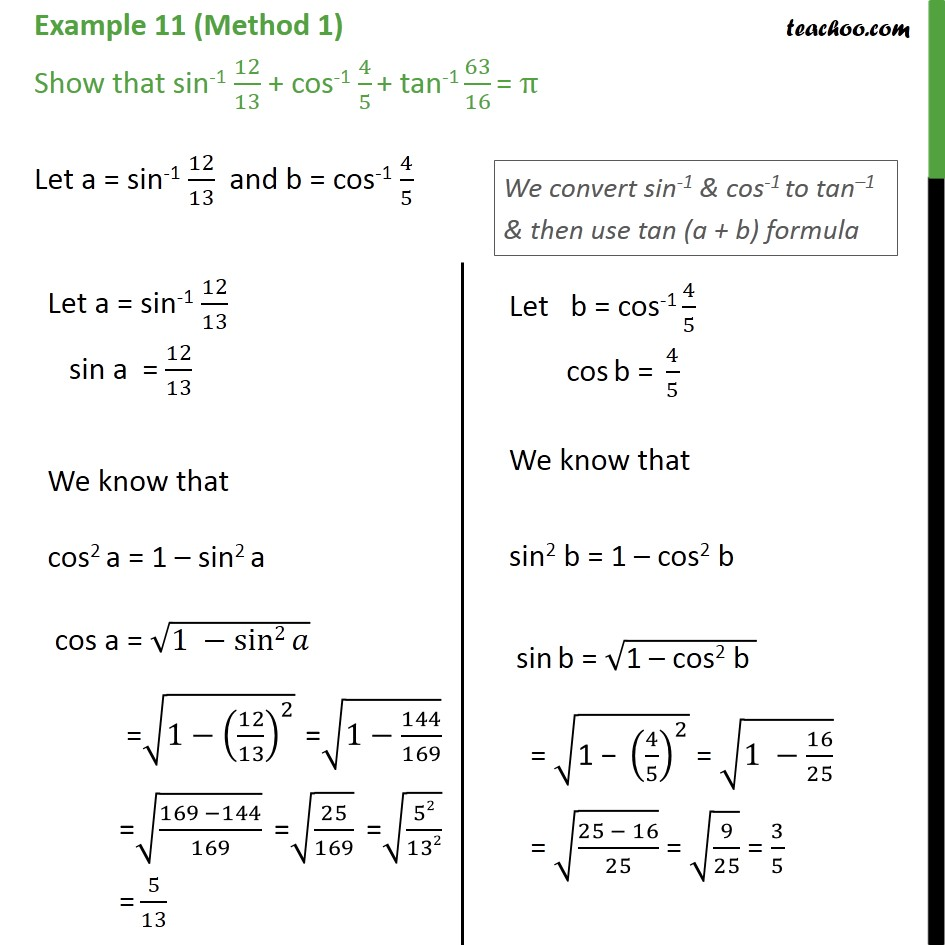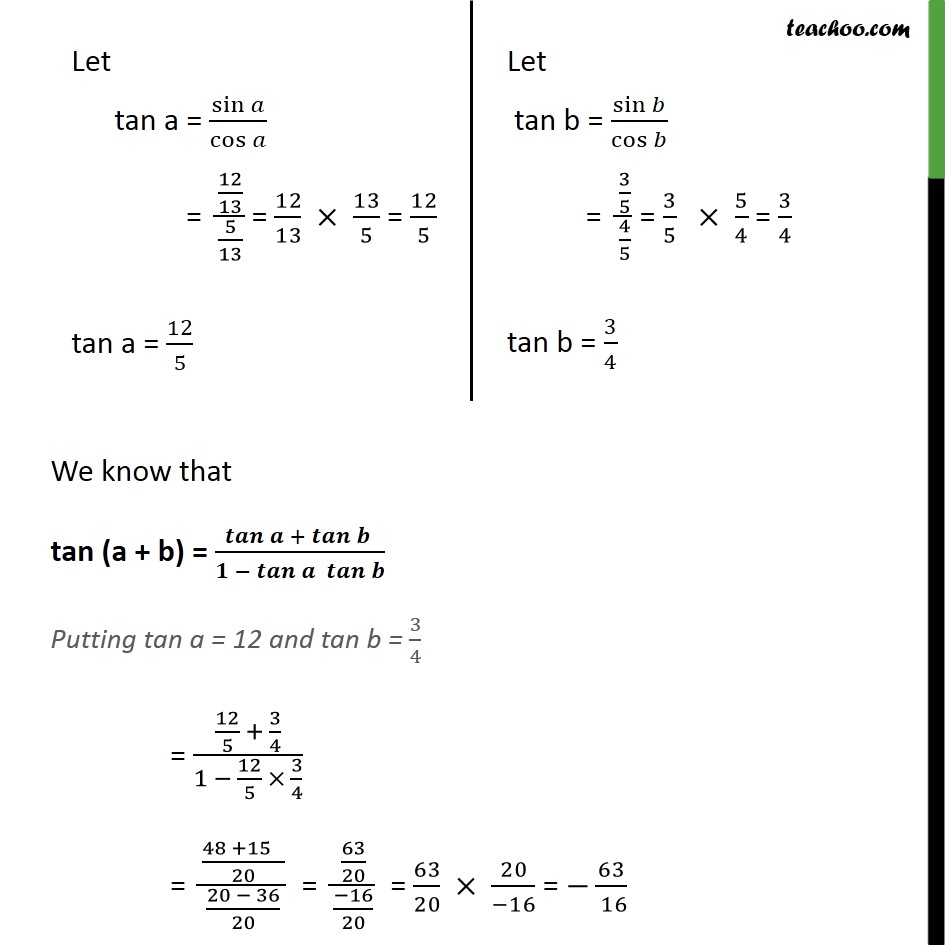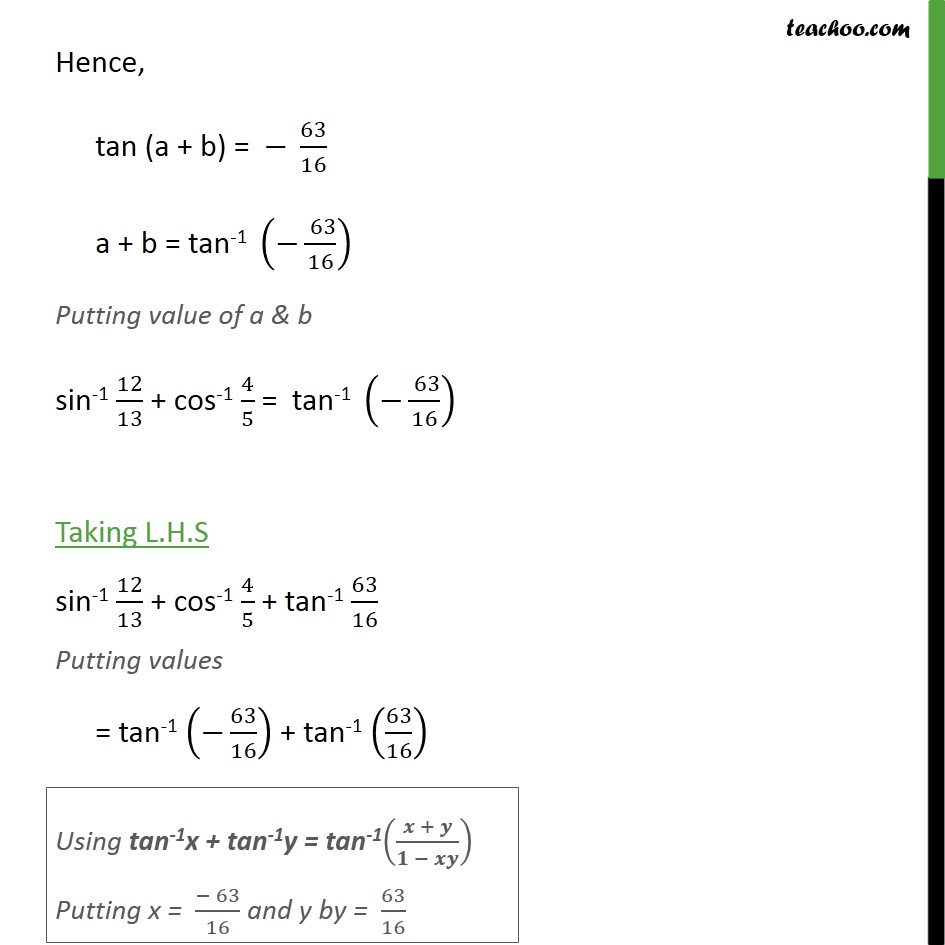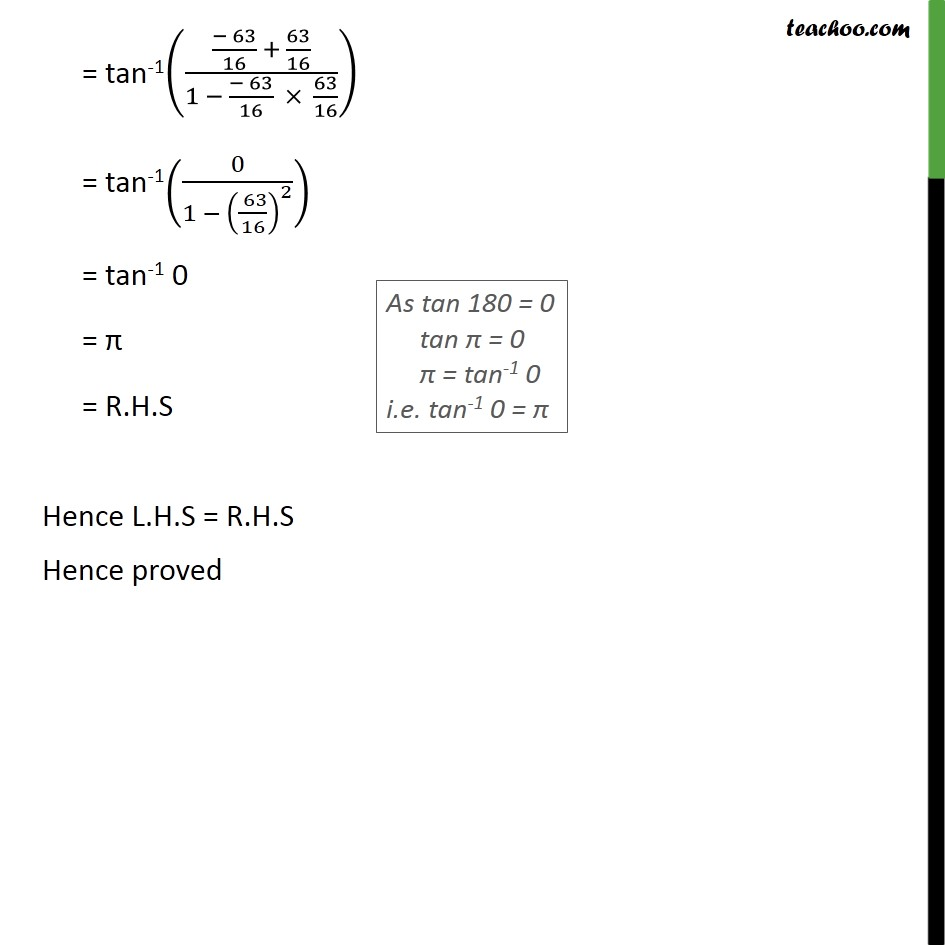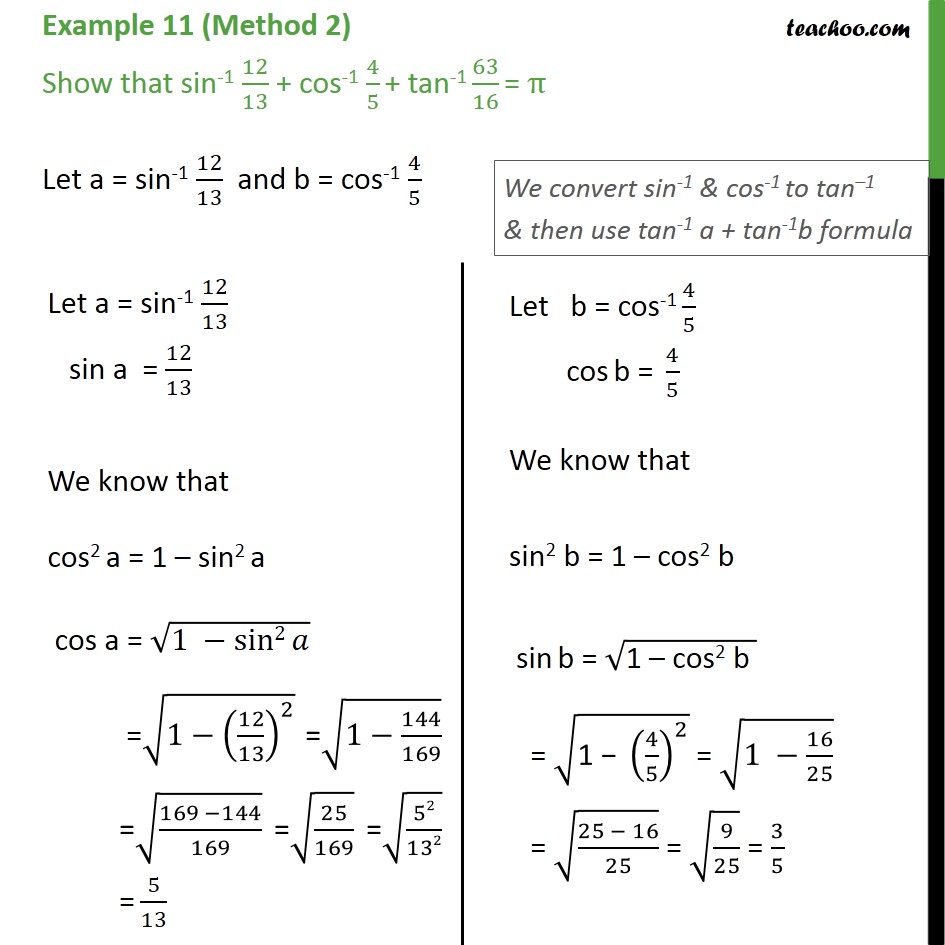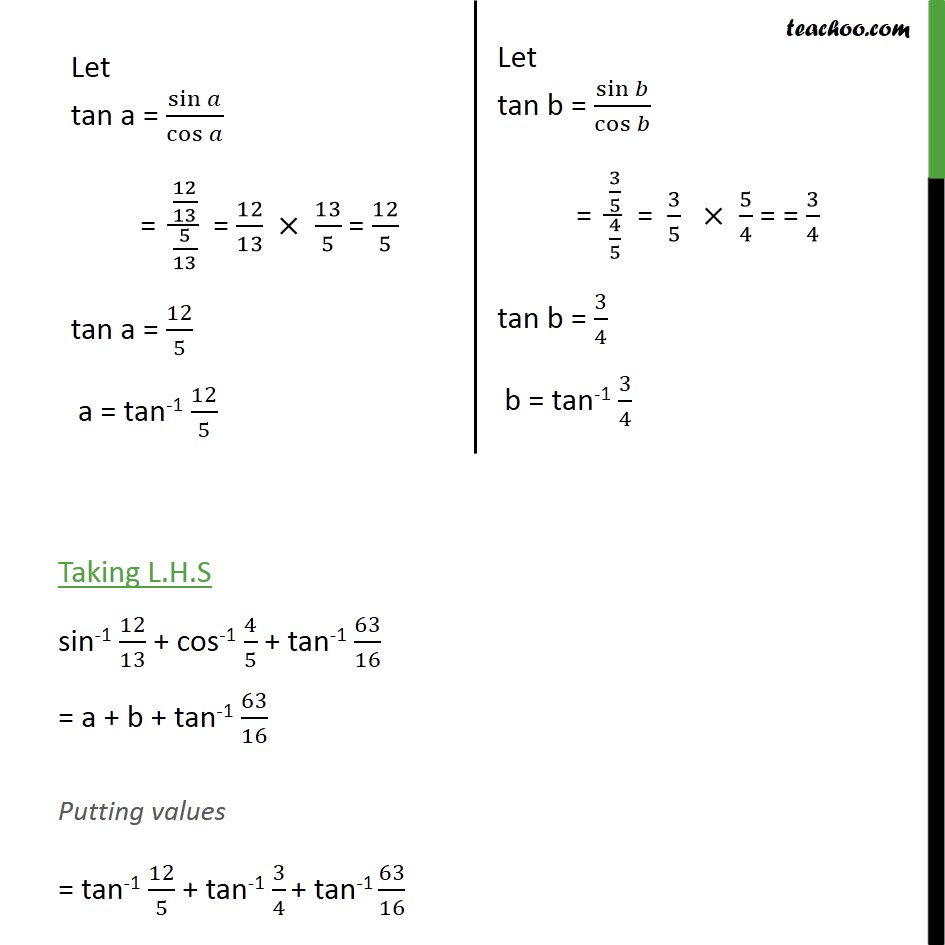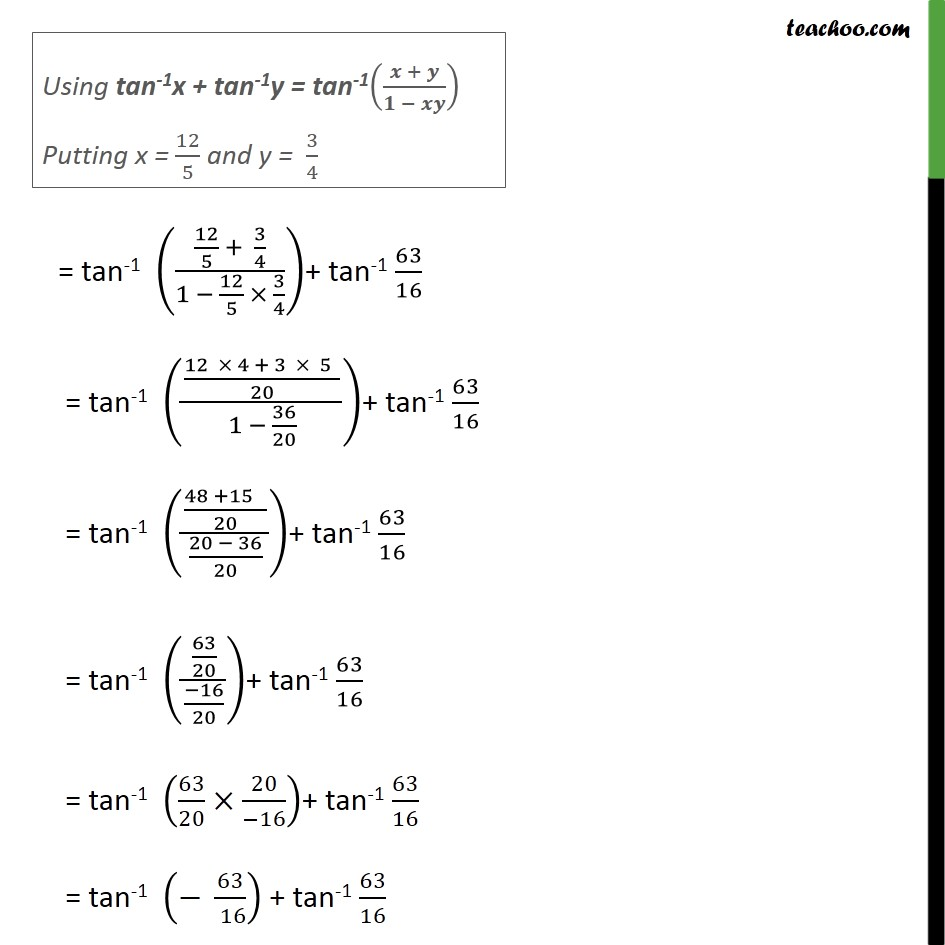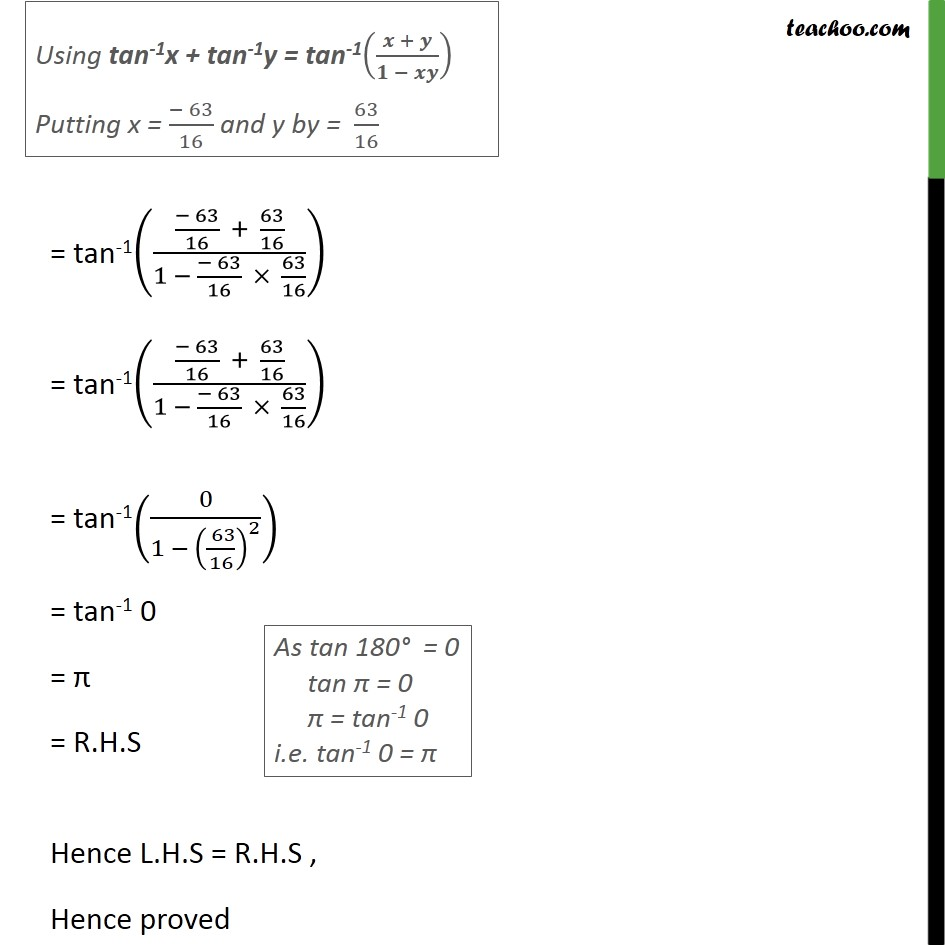1. Chapter 2 Class 12 Inverse Trigonometric Functions
2. Serial order wise
3. Examples

Transcript

Example 11 - Chapter 2 Class 12 Inverse Trigonometric Functions - NCERT Book (Method 1) Show that sin-1 12/13 + cos-1 4/5 + tan-1 63/16 = π Let a = sin-1 12/13 and b = cos-1 4/5 We convert sin-1 & cos-1 to tan–1 & then use tan (a + b) formula Let a = sin-1 12/13 sin a = 12/13 We know that cos2 a = 1 – sin2 a cos a = √(1 −sin2⁡𝑎 ) =√(1−(12/13)^2 ) " =" √(1−144/169) " =" √((169 −144)/169) " =" √(25/169) " =" √(52/132) "=" 5/13 Let tan a = sin⁡𝑎/cos⁡𝑎 = (12/13)/(5/13) = 12/13 × 13/5 = 12/5 tan a = 12/5 Let b = cos-1 4/5 cos b = 4/5 We know that sin2 b = 1 – cos2 b sin b = √("1 – cos2 b " ) = √("1 − " (4/5)^2 ) = √(1 −16/25) = √((25 − 16)/25) = √(9/25) = 3/5 Let tan b = sin⁡𝑏/cos⁡𝑏 = (3/5)/(4/5) = 3/5 × 5/4 = 3/4 tan b = 3/4 We know that tan (a + b) = 𝒕𝒂𝒏⁡〖𝒂 +〖 𝒕𝒂𝒏〗⁡〖𝒃 〗 〗/(𝟏 − 𝒕𝒂𝒏⁡〖𝒂 𝒕𝒂𝒏⁡𝒃 〗 ) Putting tan a = 12 and tan b = 3/4 = (12/5 + 3/4)/(1 − 12/5 × 3/4) = ((48 +15 )/20)/((20 − 36)/20) = (63/20)/((−16)/20) = 63/20 × 20/(−16) = −63/( 16) Hence, tan (a + b) = − 63/16 a + b = tan-1 (−( 63)/16) Putting value of a & b sin-1 12/13 + cos-1 4/5 = tan-1 (−( 63)/16) Taking L.H.S sin-1 12/13 + cos-1 4/5 + tan-1 63/16 Putting values = tan-1 (−63/16) + tan-1 (63/16) Using tan-1x + tan-1y = tan-1((𝒙 + 𝒚)/(𝟏 − 𝒙𝒚)) Putting x = (− 63)/16 and y by = 63/16 = tan-1(((− 63)/16 + 63/16)/(1 − (− 63)/16 × 63/16)) = tan-1(0/(1 − (( 63)/16)^2 )) As tan 180 = 0 tan π = 0 π = tan-1 0 i.e. tan-1 0 = π = tan-1 0 = π = R.H.S Hence L.H.S = R.H.S Hence proved Example 11 (Method 2) Show that sin-1 12/13 + cos-1 4/5 + tan-1 63/16 = π Let a = sin-1 12/13 and b = cos-1 4/5 We convert sin-1 & cos-1 to tan–1 & then use tan-1 a + tan-1b formula Let a = sin-1 12/13 sin a = 12/13 We know that cos2 a = 1 – sin2 a cos a = √(1 −sin2⁡𝑎 ) =√(1−(12/13)^2 ) " =" √(1−144/169) " =" √((169 −144)/169) " =" √(25/169) " =" √(52/132) "=" 5/13 Let tan a = sin⁡𝑎/cos⁡𝑎 = (12/13)/(5/13) = 12/13 × 13/5 = 12/5 tan a = 12/5 a = tan-1 𝟏𝟐/𝟓 Let b = cos-1 4/5 cos b = 4/5 We know that sin2 b = 1 – cos2 b sin b = √("1 – cos2 b " ) = √("1 − " (4/5)^2 ) = √(1 −16/25) = √((25 − 16)/25) = √(9/25) = 3/5 Let tan b = sin⁡𝑏/cos⁡𝑏 = (3/5)/(4/5) = 3/5 × 5/4 = = 3/4 tan b = 3/4 b = tan-1 𝟑/𝟒 Taking L.H.S sin-1 12/13 + cos-1 4/5 + tan-1 63/16 = a + b + tan-1 63/16 Putting values = tan-1 12/5 + tan-1 3/4 + tan-1 63/16 Using tan-1x + tan-1y = tan-1((𝒙 + 𝒚)/(𝟏 − 𝒙𝒚)) Putting x = 12/5 and y = 3/4 = tan-1 ((12/5 + 3/4)/(1 − 12/5 × 3/4))+ tan-1 63/16 = tan-1 (((12 × 4 + 3 × 5 )/20)/(1 − 36/20))+ tan-1 63/16 = tan-1 (((48 +15 )/20)/((20 − 36)/20))+ tan-1 63/16 = tan-1 ((63/20)/((−16)/20))+ tan-1 63/16 = tan-1 (63/20×20/(−16))+ tan-1 63/16 = tan-1 (− 63/( 16)) + tan-1 63/16 Using tan-1x + tan-1y = tan-1((𝒙 + 𝒚)/(𝟏 − 𝒙𝒚)) Putting x = (− 63)/16 and y by = 63/16 = tan-1(((− 63)/16 + 63/16)/(1 − (− 63)/16 × 63/16)) = tan-1(((− 63)/16 + 63/16)/(1 − (− 63)/16 × 63/16)) = tan-1(0/(1 − (( 63)/16)^2 )) As tan 180° = 0 tan π = 0 π = tan-1 0 i.e. tan-1 0 = π = tan-1 0 = π = R.H.S Hence L.H.S = R.H.S , Hence proved

Examples